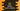# Dart check for any or every element in a list satisfy a given condition## Dart check for any or every element in a list satisfy a given condition:

In this post, we will learn how to check if any one or every elements in a list satisfies a given condition or not. Dart list provides two methods called any() and every() that can be used to solve this easily. We can also use a loop to do that. I will show you both of these approaches in this article i.e. by using a for in loop and by using inbuilt methods.

### Method 1: Check if any of the elements in a list satisfy a condition using loop:

Let’s use one for in loop to solve it:

``````void main() {
var givenList = [1, 2, 3, 4, 5, -1, 2, 9];

var found = false;

for (var item in givenList) {
if (item < 0) {
found = true;
break;
}
}

if (found) {
print("This list contains element smaller than zero");
} else {
print("This list doesn't contain any element smaller than zero");
}
}``````
• In this program, we are using one for in loop to iterate over the list elements one by one.
• found variable is initialized as false. This variable is changed to true if any element smaller than zero is found in the list.
• If the program find any item less than the current value inside the loop, it changes found to true and breaks from the loop.
• Once the loop ends, we are checking the value of found and printing one message based on its value.

If you run the above program, it will print the below message:

``This list contains element smaller than zero``

If you remove -1 from the list and run it again, it will print the second message.

### Method 2: Check if any of the elements in a list satisfy a condition using any():

Using any is easy than using a loop. It returns one boolean value if any element in the list satisfies a condition.

``````void main() {
var givenList = [1, 2, 3, 4, 5, -1, 2, 9];

var found = givenList.any((e) => e < 0);

if (found) {
print("This list contains element smaller than zero");
} else {
print("This list doesn't contain any element smaller than zero");
}
}``````

Based on the returned boolean, we can print the result.

### Method 3: Check if every element of a list satisfy a condition using a loop:

To check if all elements of a list satisfies a condition or not, we can use one loop as like below:

``````void main() {
var givenList = [1, 2, 3, 4, 5, -1, 2, 9];

var flag = true;

for (var item in givenList) {
if (item < 0) {
flag = false;
break;
}
}

if (flag) {
print("All items are smaller than 0");
} else {
print("Not all items are smaller than 0");
}
}``````

Here, we are using one boolean value flag to indicate if all elements are less than 0 or not.

### Method 4: Check if every element of a list satisfy a condition using every:

every() can be used instead of for in loop to do the same thing in just one line:

``````void main() {
var givenList = [-1, -2, -3, -4, -5, -1, -2, -9];

var flag = givenList.every((e) => e<0);

if (flag) {
print("All items are smaller than 0");
} else {
print("Not all items are smaller than 0");
}
}``````

So, it is just one line that can give us the result. It returns one boolean value defining every elements of the list satisfy a given condition or not. Based on this boolean value, we can say if all items satisfy that condition or not. For the above example, we are checking if all elements are less than 0 or not.